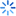• 签到
•苹果/安卓/wp

•苹果/安卓/wp

客户端

# 想问下，R语言用什么函数来对cox回归做逐步法。

2018/04/07 14:28

## 满意回答

2018/04/15 18:13

n = 10000
beta1 = 2; beta2 = -1
lambdaT = .002 # baseline hazard
lambdaC = .004  # hazard of censoring

x1 = rnorm(n,0)
x2 = rnorm(n,0)
# true event time
T = rweibull(n, shape=1, scale=lambdaT*exp(-beta1*x1-beta2*x2))
C = rweibull(n, shape=1, scale=lambdaC)  #censoring time
time = pmin(T,C)  #observed time is minof censored and true
event = time==T   # set to 1 if event isobserve

0人关注该问题
+1加载中...